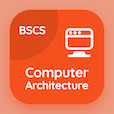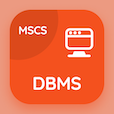Online CS Degree Courses

Digital Logic Design Quizzes

Digital Logic Design Quiz - Complete

# Analysis of Asynchronous Sequential Logic MCQ Questions PDF Download - 77

Study Analysis of Asynchronous Sequential Logic Multiple Choice Questions and Answers (MCQs), analysis of asynchronous sequential logic quiz answers PDF, test 77 to download Digital Logic Design book. Asynchronous Sequential Logic MCQ trivia questions, analysis of asynchronous sequential logic Multiple Choice Questions (MCQ Quiz) for online college degrees. Analysis of Asynchronous Sequential Logic Book PDF: binary numbers, qualifying symbols, subtraction with complement, adder and subtractors, analysis of asynchronous sequential logic test prep for computer software engineer.

"The x variable in the analysis procedure is used for" Quiz PDF: analysis of asynchronous sequential logic App APK with columns, rows, matrix, and both a and b choices for computer science programs. Learn asynchronous sequential logic questions and answers to improve problem solving skills for cheapest online computer science degree.

## Analysis of Asynchronous Sequential Logic MCQ Questions

MCQ: The x variable in the analysis procedure is used for

rows
columns
matrix
both a and b

MCQ: When the mode of adder-subtractor is 0 it will give

A-B
A+B
A/B
A*B

MCQ: Using 10's complement, subtracting 72532 from 3250 is equal to

-69272
-69282
-69252
-69232

MCQ: Magnitude comparator is represented by the qualifying symbol of

MAG
MUL
COMP
CTR

MCQ: Product of 1011 and 101

110111
110011
111011
111100

### More Quizzes from Digital Logic Design Book